Next: Gravitational potential Up: Newtonian gravity Previous: Newtonian gravity

# Introduction

Classical gravity, which is invariably the dominant force in celestial dynamical systems, was first correctly described in Newton's Principia. According to Newton, any two point objects exert a gravitational force of attraction on each other; this force is directed along the straight line joining the objects, is directly proportional to the product of their masses, and inversely proportional to the square of the distance between them. Consider two point objects of mass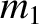and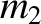that are located at position vectors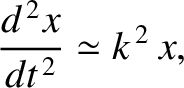and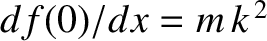, respectively. The gravitational force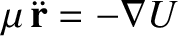that massexerts on massis written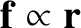(3.1)

The gravitational force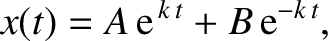that massexerts on massis equal and opposite; that is,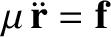. (See Figure 2.3.) Here, the constant of proportionality,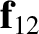, is called the universal gravitational constant and takes the value (Yoder 1995)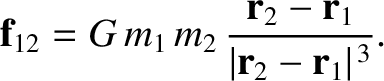(3.2)

Incidentally, there is something rather curious about Equation (3.1). According to this law, the gravitational force acting on a given object is directly proportional to that object's inertial mass. Why, though, should inertia be related to the force of gravity? After all, inertia measures the reluctance of a given body to deviate from its preferred state of uniform motion in a straight line, in response to some external force. The preceding question perplexed physicists for many years, but was eventually answered when Albert Einstein published his general theory of relativity in 1916. According to Einstein, inertial mass acts as a sort of gravitational charge because it is impossible to distinguish an acceleration produced by a gravitational field from an apparent acceleration generated by observing motion in a non-inertial reference frame. The assumption that these two types of acceleration are indistinguishable leads directly to all of the strange predictions of general relativity; for instance, clocks in different gravitational potentials run at different rates, mass bends space, and so on (Rindler 1977).Next: Gravitational potential Up: Newtonian gravity Previous: Newtonian gravity
Richard Fitzpatrick 2016-03-31